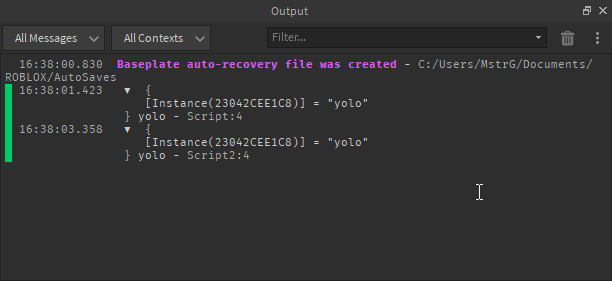# Passing Tables to another script through bindable functions causes it to change if it includes instances

You can write your topic however you want, but you need to answer these questions:

1. What do you want to achieve? Keep it simple and clear!
I want to pass tables to another script.

2. What is the issue? Include screenshots / videos if possible!
When I try to use a bindable function to pass the table, the instances in the table change.
script1:

script2:

Output:

1. What solutions have you tried so far? Did you look for solutions on the Developer Hub?
Storing the table in a module script, but I put the code to create the table in a regular script and I can’t move the table over to the module script.
2 Likes

This behavior is normal.

What you could try to do is instead of passing an instance, you could use the instance’s full name (which returns a string) and then run a function (taken from here) that will try to find the instance using the full name. This is the function:

``````local function GetInstance(String)
local Table = string.split(String, ".")
local Service = game:GetService(Table)

local ObjectSoFar = Service
for Index, Value in pairs(Table) do
if Index ~= 1 then -- Don't run over the service.
local Object = ObjectSoFar:FindFirstChild(Value)
if Object then
ObjectSoFar = Object
else
break
end
end
end

return (ObjectSoFar ~= Service and ObjectSoFar) or nil
end

-- GetInstance("Workspace.Baseplate") would return the actual baseplate
``````
2 Likes

What’s the reason you can’t move the table to a module script? I just tested this in a new place and it worked going from script to script with a module script.Source
``````--same script, Script2 just doesn't have the mod[workspace.Baseplate] line
local mod = require(script.Parent.ModuleScript)
mod[workspace.Baseplate] = "yolo"
print(mod, mod[workspace.Baseplate])
``````
3 Likes

You can use a module that implements `BindableFunction` instead of a bindable instance to circumvent this problem too.

``````-- LuaBindableFunction.lua

local BindableFunction = {
All = {}
}

function BindableFunction.new(name)
local self = {
Name = name,
Invoke = nil
}

BindableFunction.All[name] = self

return self
end

function BindableFunction.get(name)
return BindableFunction.All[name] or BindableFunction.new(name)
end

function BindableFunction.remove(name)
BindableFunction.All[name] = nil
end

return BindableFunction
``````
``````-- script1.server.lua

local BindableFunction = require(path.to.LuaBindableFunction)

local instance = workspace.Baseplate
local table = {}
table[instance] = "random data about instance here"

local bindable = BindableFunction.get("ScriptFunction")
bindable.Invoke = function()
print(table)
return table
end
``````
``````-- script2.server.lua

local BindableFunction = require(path.to.LuaBindableFunction)

wait(3)

local bindable = BindableFunction.get("ScriptFunction")
local table = bindable.Invoke()
print(table)
print(table[workspace.Baseplate])
``````

I would definitely just return a table in a module if I wanted multiple scripts to access the same table though, which is what @MrLonely1221 is suggesting.

2 Likes

Thanks everyone! I didn’t think about sending the table over to the module script by requiring it and then adding the table to the module script.